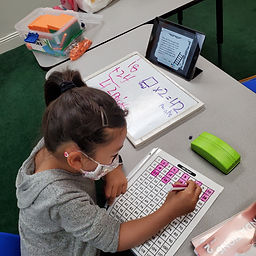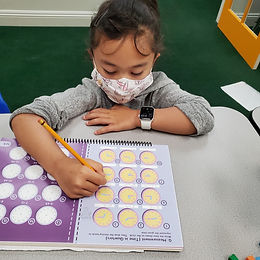Ms. Raejean

Target 1​

Lesson Type:

Review

Number Operation

:

Computation

Solve multi-step word problems within 100 (addition, subtraction, multiplication, and division).

1:

Identify the correct arithmetic processes based on the information presented in word problems.

2:

Find the key words in word problems that indicate the correct arithmetic process to use.

3:

Find and use the needed information in a word problem in order to solve.

4:

Write an equation from a word problem with a variable included in the equation. Then solve for the variable.

3rd

Vocabulary:

Activities:

Students solved multi-step word problems. Students made sense of the problem, underlined the question, and circled important information, including key words like combined. Students rephrased in their own words what needed to be solved. Students determined the operations to be used and wrote equations. Then students used an appropriate strategy to solve the problem. Example - use base ten blocks to subtract or skip counting on the hundreds chart to multiply and divide. After solving the problem, students practiced writing the solution with a label or in a sentence. *Writing an equation with an unknown number was introduced.Home Exploration

Guiding Questions:Absent Students:

Target 2

:

1:

Recognize that a time on the hour will have the minute hand on the 12 on an analog clock and 00 on the digital clock.

2:

Recognize that a time on the half-hour will have the minute hand on the 6 on an analog clock and 30 on the digital clock.

3:

Learn the meaning of time expressions such as half-past, quarter-past, and quarter-to.

3rd

Vocabulary:

Analog, Hour, Minute, Half Past, Quarter To, Quarter Past

Activities:

Students practiced reading analog clocks to tell time in half-hours and quarters. We talked about looking at the position of the hour hand to determine the appropriate hour. We worked on using the phrases Half Past, Quarter To, and Quarter Past, but we will continue to practice.Home Exploration

Guiding Questions:Target 3

:

Vocabulary:

Activities:Home Exploration